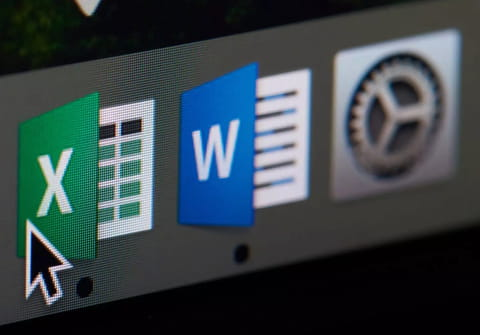# Copy data from one Excel workbook to anotherThis article will teach you how to copy or transfer data between spreadsheets in Microsoft Excel using VBA. In this particular example, we will also explain how to combine data by using an append query. This combination of tasks will allow you to combine data in existing worksheets for easier analysis.

## How to make a macro to move or copy data in Excel?

Let's take a case in which you need to copy data from one workbook and then amend the content to another workbook. In this example, workbook one (the source workbook) has 1 2 3 4 5 and workbook two has 6 7 8 9 0.

After running the macro, workbook two should have 6 7 8 9 0 1 2 3 4 5. The formats of both workbooks is the same.

Here is a macro that could transfer and append your data. (Make sure that you read the NOTE in the code):

```Sub CopyData()
Dim sBook_t As String
Dim sBook_s As String
Dim sSheet_t As String
Dim sSheet_s As String
Dim lMaxRows_t As Long
Dim lMaxRows_s As Long
Dim sMaxCol_s As String
Dim sRange_t As String
Dim sRange_s As String
sBook_t = "Target Data WB- Copy data to WB.xls"
sBook_s = "Source Data WB - Copy data to WB.xls"
sSheet_t = "Target WB"
sSheet_s = "Source"
lMaxRows_t = Workbooks(sBook_t).Sheets(sSheet_t).Cells(Rows.Count, "A").End(xlUp).Row
lMaxRows_s = Workbooks(sBook_s).Sheets(sSheet_s).Cells(Rows.Count, "A").End(xlUp).Row
sMaxCol_s = Mid(sMaxCol_s, 2, InStr(2, sMaxCol_s, "\$") - 2)
If (lMaxRows_t = 1) Then
sRange_t = "A1:" & sMaxCol_s & lMaxRows_s
sRange_s = "A1:" & sMaxCol_s & lMaxRows_s
Workbooks(sBook_t).Sheets(sSheet_t).Range(sRange_t) = Workbooks(sBook_s).Sheets(sSheet_s).Range(sRange_s).Value
Else
sRange_t = "A" & (lMaxRows_t + 1) & ":" & sMaxCol_s & (lMaxRows_t + lMaxRows_s - 1)
sRange_s = "A2:" & sMaxCol_s & lMaxRows_s
Workbooks(sBook_t).Sheets(sSheet_t).Range(sRange_t) = Workbooks(sBook_s).Sheets(sSheet_s).Range(sRange_s).Value
' ###################### NOTE #################
'the following lines are to be used of serial number is to be fixed too, instead of being copied
' if there is no need, then delete the line below
Workbooks(sBook_t).Sheets(sSheet_t).Range("A" & lMaxRows_t).AutoFill Destination:=Workbooks(sBook_t).Sheets(sSheet_t).Range("A" & lMaxRows_t & ":A" & (lMaxRows_t + lMaxRows_s - 1)), Type:=xlFillSeries
End If
End Sub```
Any more excel questions? check out our forum!
Subject Replies
Around the same subject